## 41) Bonds

Ten Plus Bonds: Three In a Row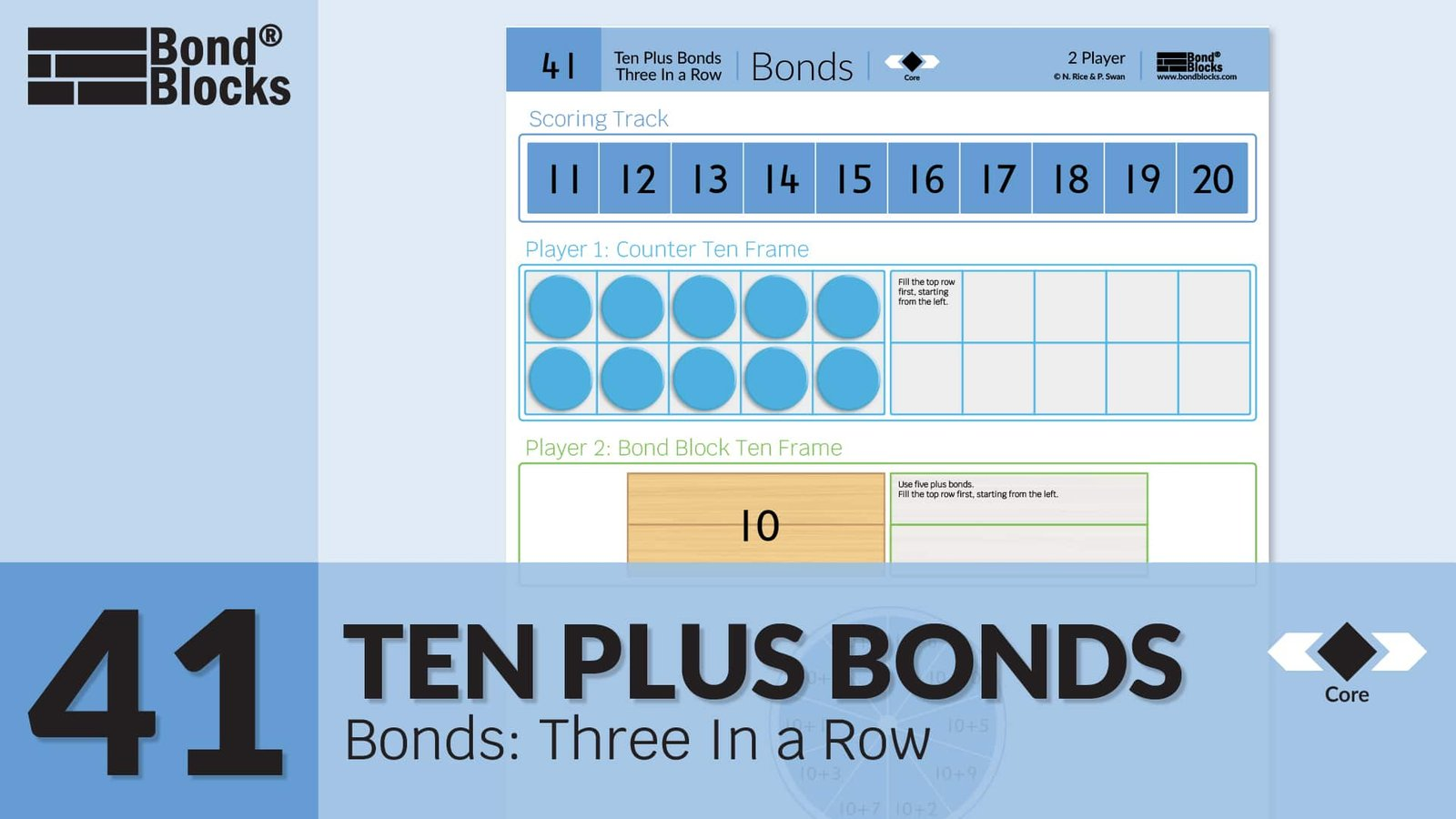### Mathematics

• Develop the concept of numbers between 10 and 20 as a ten plus bond, ten plus another amount of ones.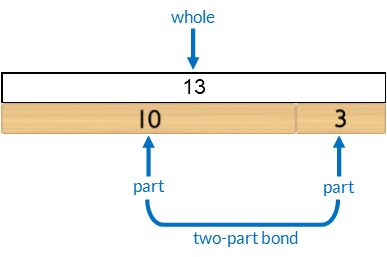• Develop fluency sequencing numbers between 10 and 20.

### Language

•  ten plus bond: a bond of ten and one other part makes the whole.
• equals
• before, after
• greater, less

### Core Activity Observations

##### Language “teen” verses “ty”

Listen carefully to students’ pronunciation of “teen”. For example, “fourteen”.

• The “teen” means ‘add one ten’.  For example, “fourteen” is ‘four add one ten’.
• This is different to “ty” which means groups of ten. For example, “forty” is four tens.

Assist students who say ‘teen’ or ‘ty’ incorrectly by completing the following activities. The aim of these activities is to help students:

• Make correct mathematical connections between the number said, written number and quantity (Bond Blocks).
• Understand and use the meaning of mathematical suffixes correctly (endings to words).
##### “teen” activity

An activity for individual students. You will need:

• One linear 10 block.
• One of each Bond Block from 3 to 9, placed within reach of the student.
• One pencil.

Instruct the student to:

• Point to it the number “thirteen” on the Number Board.
• Say the number.
• Make this number with Bond Blocks. Then place the Bond Blocks on top of the Number Board.

Repeat for each teen (i) Point to the number on the Number Board, (ii) Say it, (iii) Make it with Bond Blocks and place them on the number board. Students will only need to replace the ones block each time a new number is made.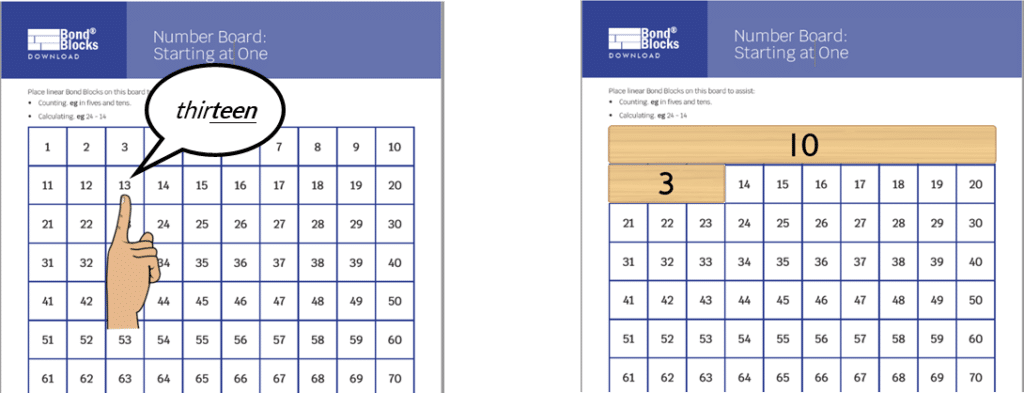Remove all the Bond Blocks from the Number Board.

• Instruct the student to underline the one, worth 10, in each teen number on the board.
• Define “teen” as add ten.
• Instruct the student to point to each number, saying them correctly.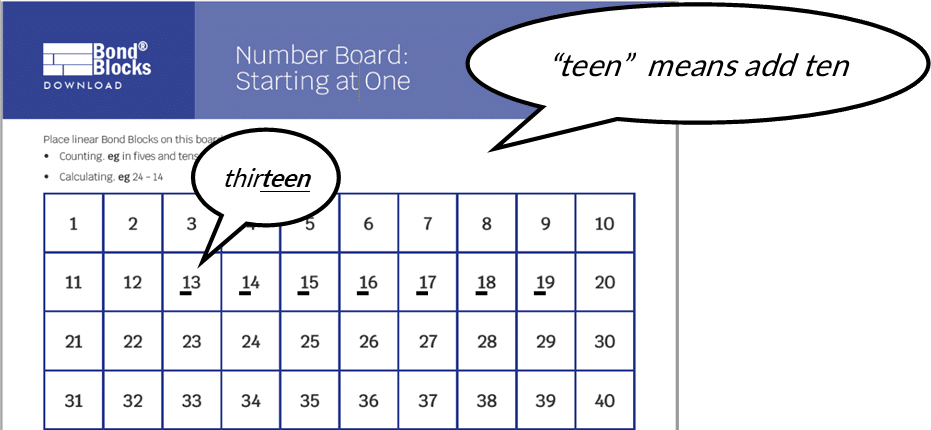##### “ty” activity

An activity for individual students. You will need:

• Two sets of Bond Blocks. This will be 8 linear 10 blocks. The remaining 10 can be build with two blank fives.
• One pencil.
Instructions:
• The teacher models pointing to each number, from 10 to 90, counting aloud. Emphasize the “ty”.
• The student repeats counting and pointing.
• The teacher models placing each block on the Number Board, counting aloud by tens, from 10 to 90, as each block is placed. Emphasize the “ty”.
• The student to repeats counting whilst placing the blocks.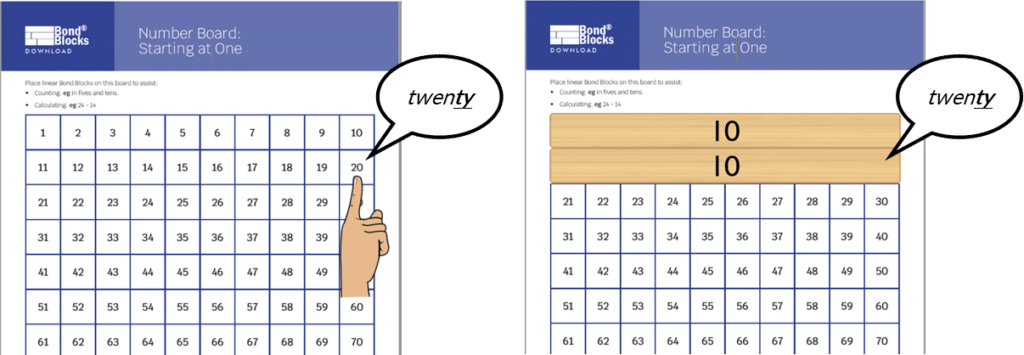Remove all the Bond Blocks from the Number Board.

• Instruct the student to underline the zero, worth groups of ten, in each number on the board from 10 to 90.
• Define “ty” as groups of ten.
• Instruct the student to point to each number, saying them correctly.##### Persistent incorrect language

If students persist in saying “teen” or “ty” incorrectly it can be helpful to record this and play it back to the student for them to identify their error.

• The teacher points to the number, on the Number Board, that the student says incorrectly.
• Instruct the student to make this amount with Bond Blocks. It is important the student makes the number with Bond Blocks first to ensure the misunderstanding is only in saying the number, not in representing the quantity.
• Tell the student you are going to record them saying the number. Instruct the student to say the number. Record the student as they say the number incorrectly.
• Tell the student they are saying the number incorrectly. Say the number aloud to the student, correctly, emphasizing the correct suffix.
• Play back the recording of the student incorrectly saying the number, asking the student to listen and identify what they said incorrectly.

Complete both  the “teen” and “ty” activities (outlined above the Persistent Incorrect Language section).

Then reassess the student. Instruct them to:

• Point to 14 on the Number Board, make this with Bond Blocks, then say the number.
• Point to 40 on the Number Board, make this with Bond Blocks, then say the number.## Differentiation

### A little easier

##### Sequencing cards

Click to download Ten Plus Bonds: Bonds Sequencing Numbers to 20 Cards.

Cut out the cards. Randomise the order within each set of cards (hands, empty ten frames, numbers) to complete the following activities.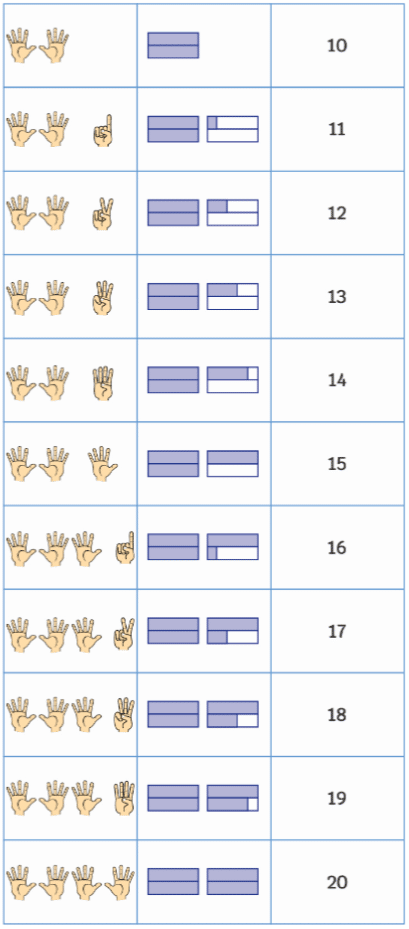Complete each of these activities with one set of cards. For example, hand cards. Then repeat it with the other sets before moving to the next activity.

1. Counting Forwards
• Sequence the cards in counting order.
• Point to each card as the number is said.
• Identify place value patterns in each column and row.

2. Counting backwards

• Start with the 20 card. Sequence the cards counting backwards to 10.
• Point to each card as the number is said.

3. Before and After

• Turn all cards in one set face down. Turn over one card.
• Say the number one before (one less than). Turn this card over to confirm.
• Say the number one after (one more than). Turn this card over to confirm.
• Turn these three cards face over and repeat with a different card from that set.
##### Directional difficulties

Mathematics is different to English. When teaching reading English fluency moving from left to right is stressed. Mathematics requires students to be fluent moving in many directions. Consider:

• Horizontal movement on a number line. Move right to count forwards, add,  and increase the value of numbers.  Move left to count backwards, subtract by taking away, and reduce the value of numbers.
• The place value chart is opposite to this. Values increase to the left and decrease to the right.
• Addition and subtraction strategies. When adding and subtracting mentally it is often easier to use place value and work from left to right. However, when adding, subtracting and multiplying using an algorithm calculations are completed from right to left. The division algorithm is completed in the opposite direction.
• Vertical movement on a number line as per a thermometer or y-axis on a graph.
• Diagonal and curved movement as per a line or parabola.
• Rotations clockwise and anticlockwise.

Click to download a Comparison and Number line Language: Desk Visual to support students who have directional and sequencing difficulties.##### Fluency card games

Use all the cards from the Ten Plus Bonds: Bonds Sequencing Numbers to 20 Cards, including Wild Cards, to make one deck.

• Play Memory. Arrange the cards in two arrays: one of the number cards and the other of ten frame cards. Adjust the number of cards included to the level of the students.
• Play Go Fish. Deal five cards each and collect pairs. The Wild Cards represent any value.
• Play Guess My Number. Place the cards in a shuffled pile face down. Player One draws the top card, looks at it without showing anyone. Player One gives clues so as the other player can guess the card drawn. Model language of tens, ones, greater than, less than, odd, even.
• Play Snap. Students must say the number of the card as it is placed. The Wild Card can be any value. A littler harder is Snap +/- One.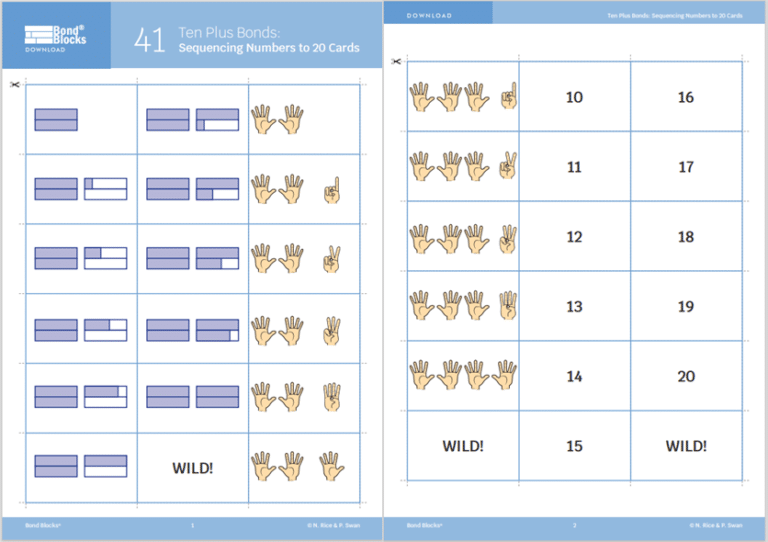### A little harder

##### Sequence cards using even and odd numbers

Cut out the cards from the Ten Plus Bonds: Bonds Sequencing Numbers to 20 Cards.  Randomise the order within each set of cards (hands, empty ten frames, numbers) to complete the following activities. Complete each of these activities with one set of cards. For example, hand cards. Then repeat it with the other sets before moving to the next activity.

Even numbers:

• Sequence the cards counting forwards, by two using even numbers. Start at ten.
• Repeat counting back by two. Start from 20.
• Turn the cards face down. Turn over one card. Say the number before (two less) and after this (two greater). Turn the cards to confirm.Odd numbers:

• Sequence the cards counting forwards, by two using odd numbers. Start from eleven.
• Repeat counting back by two. Start from 19.
• Turn the cards face down. Turn over one card. Say the number before (two less) and after this (two greater). Turn the cards to confirm.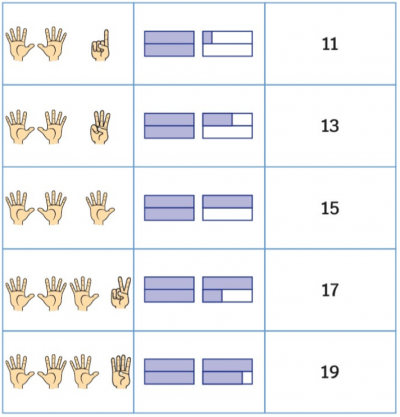### Progression

In the next activity students represent ten plus bonds using a variety of manipulatives. Go to

##### Activity 42

Ten Plus Bonds: Bonds, Multiple Representations